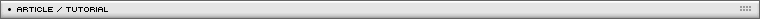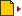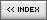Rotation around a 3d (x,y,z) axis
[ July 13, 2004 ] by Eric Lin
Basic 3D in Flash: 4/5
In this fourth article, the author talks about rotating 3D points around an arbitrary axis.

I have seen equations that rotate 3d data around a 3d (x,y,z) axis. I don't understand that complex equations. Anyway, I made them mine. Less effective but reasonable.

To rotate data around x or y or z axis is easy. For example, we rotate (x,y,z) around Z axis, then we can ignore z value and take (x,y) in 2D. To rotate (x,y) in 2D for an arc angle delta, we just convert (x,y) data into radius and angle. The radius is the distance (x,y) to (0,0); that is Math.sqrt(x*x+y*y); The angle arc in PI format is Math.atan2(y,x); now rotate a delta angle. The radius is the same. While the angle should increases by delta. So, the new (x,y) is x=radius*Math.cos(angle+delta); y=radius*Math.sin(angle+delta).

```function rotateZ(x, y, center, dAngle)
{
//note: here dAngle is in arc unit PI format
var dy = y-center.y;
var dx = x-center.x;
var orgAngle = Math.atan2(dy, dx);
var hypo = Math.sqrt(dy*dy+dx*dx);
var newAngle = orgAngle+dAngle;
var xx = hypo*Math.cos(newAngle)+center.x;
var yy = hypo*Math.sin(newAngle)+center.y;
var pt = {x:xx, y:yy};
return pt;
}```

The same math equation can be modified easily to take (z,x) to rotate around Y axis, take (y,z) to rotate around X axis. But what if the axis is not straight Z, but has a little deviation? Then we can't take only (x,y), we need to handle z.

Well, I said that, I dont understand this complex math. So, I try to find a work-around. I make it by several steps. Each step is a rotate around coordinate axis. First I rotate it around Z axis, to make the axis in the YZ plane. Then I rotate it around X axis to make the axis the same as Y axis. OK, we can easily do a rotation around Y now.

After this, I counter-rotate it by the same angle as above to go off the Y axis back to the YZ plane. Then I counter-rotate the angle to make the axis back to the original (x,y,z) vector. That is it. Take a pen and paper, it should not be difficult to find out during steps what angle should be rotated.

See the movie below and click the play button to show steps.

This is the final result: